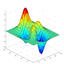Sylph 0.1.0

There is a newer version of this package available.
See the version list below for details.
Install-Package Sylph -Version 0.1.0
dotnet add package Sylph --version 0.1.0
<PackageReference Include="Sylph" Version="0.1.0" />
For projects that support PackageReference, copy this XML node into the project file to reference the package.
#r "nuget: Sylph, 0.1.0"
#r directive can be used in F# Interactive, C# scripting and .NET Interactive. Copy this into the interactive tool or source code of the script to reference the package.
// Install Sylph as a Cake Addin

// Install Sylph as a Cake Tool
#tool nuget:?package=Sylph&version=0.1.0

Sylph

Sylph (symbolic proof helper) is a language-integrated interactive theorem prover for F# which helps a user formally prove two F# functions or expressions are equivalent according to the axioms and rules of a particular proof system.

Unlike other theorem provers Sylph does not require an external DSL or parser for expressing theorem statements, or an external interactive environment for creating and storing the state of proofs. Theorems are expressed as the equivalence of 2 formulas with the same domain and co-domain and a formula is defined as any F# function of a particular type for which a code quotation and full expression tree are available. Formulas in a theorem do not have to be logical formulas but any 2 formulas where it makes sense to reason about equationally.

open Sylph

// Define some integer formulae of interest
let F1 = F (fun x -> 2 * x + 8)
let F2 = F (fun x -> 2 * x + 3 + 5)
let F3 = F (fun x -> 3 * x + 6 + 2 * x + 4)
let F4 = F (fun x -> 5 * x + 10)
// Or use a reflected definition

[<ReflectedDefinition>]
let f5 x = x * x + 4 * x

let F5 = F f5

// Each formula has a symbolic expression
F1.Expr
Lambda (x,
[Call (None, op_Multiply, [Value (2), x]), Value (8)]))
// And can also be decompiled to the F# source
F1.Src
"fun x -> 2 * x + 8"

Proofs are constructed according to the axioms and rules of a proof system which define the rules that can be used to match and transform formula expressions that preserve equivalence.

// Open the integer arithmetic proof system
open IntegerArithmetic

// Define some integer arithmetic formulae
let a = F (fun x -> 3 * x + 5)
let b = F (fun x -> 5 + 3 * x)
let c = F (fun x -> 6 * x)

//Some theorems are true axiomatically
// e.g the functions a and b are equivalent because of the commutativity axiom of integer arithmtic.
integer_arithmetic |- (a <=> b)
true

Axioms are pure functions that match patterns in primitive unary and binary formulas e.g the addition identity axiom for integer arithmetic is defined as:

function
| a1, Add(a2, Int32 0) when sequal a1 a2 -> Some true
| Lambda(_, a1), Lambda(_, Add(a2, Int32 0)) when sequal a1 a2 -> Some true
| Add(a1, Int32 0), a2 when sequal a1 a2 -> Some true
| Lambda(_, Add(a1, Int32 0)), Lambda(_, a2) when sequal a1 a2 -> Some true
| _ -> None
// True because of the addition identity axiom
integer_arithmetic |- (c <=> F(fun x -> 6*x + 0))
true

Proof systems also contain rules that are valid ways to transform two function expressions when they are not in a primitive unary or binary form. Theorems usully require a proof which is just a list of rule applications that must all be instances of rules defined only by the proof system.

// Not provable directly from axioms: 2x + 5 + 3 <=> 2x + 8
integer_arithmetic |- (F1 <=> F2)
false
// Proof of F1 <=> F2 using two steps
let p1 = proof (F1 <=> F2) integer_arithmetic [
right_assoc_b
equal_constants_a_b
]
Proof of A: fun x -> 2 * x + 8 <=> B: fun x -> 2 * x + 3 + 5:
1. B is right-associative: fun x -> 2 * x + 3 + 5 <=> fun x -> 2 * x + (3 + 5)
Proof incomplete.
2. Reduce equal constants in A and B: fun x -> 2 * x + (3 + 5) <=> fun x -> 2 * x + 8
Proof complete.

Rules are defined as recursive pure functions that preserve equivalence between two formulae e.g the rule of right associativity for arithmetic operators is implemented as:

let rec right_assoc =
function
| Add(Add(a1, a2), a3) -> <@@ %%a1 + (%%a2 + %%a3) @@>
| Subtract(Subtract(a1, a2), a3) -> <@@ %%a1 - (%%a2 + %%a3) @@>
| Multiply(Multiply(a1, a2), a3) -> <@@ %%a1 * (%%a2 * %%a3) @@>
| expr -> traverse expr right_assoc
// Apply the right_assoc rule to a formula expression and compare
F2.Expr, right_assoc F2.Expr
(Lambda (x,
[Call (None, op_Multiply, [Value (2), x]), Value (3)]),
Value (5)])),
Lambda (x,
[Call (None, op_Multiply, [Value (2), x]),
Call (None, op_Addition, [Value (3), Value (5)])])))

Rules are normal F# functions that can be chained together:

// Rules on formula expressions can be chained together.
(right_assoc >> equal_constants) F2.Expr
Lambda (x,
[Call (None, op_Multiply, [Value (2), x]), Value (8)]))
// Apply two rules and compare the resulting source
src F2.Expr, (right_assoc >> equal_constants >> src) F2.Expr
("fun x -> 2 * x + 3 + 5", "fun x -> 2 * x + 8")

In the above case we can see that the two formulae F1 and F2 are equivalent since one can be transformed into another and we use these two rules in our proof p1.

NuGet packages (1)

Showing the top 1 NuGet packages that depend on Sylph:

Sylvester.AbstractAlgebra

F# Library for defining, exploring and proving concepts in abstract algebra.

GitHub repositories

This package is not used by any popular GitHub repositories.